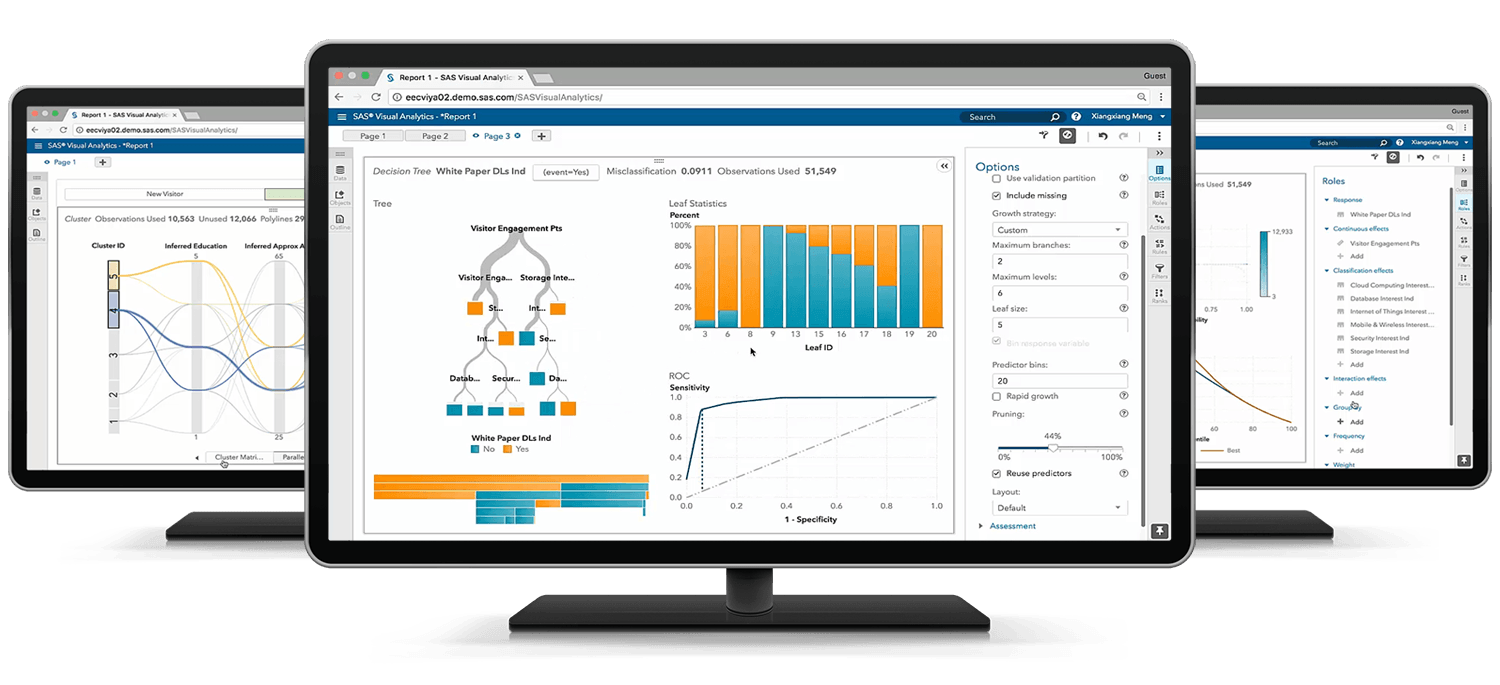# SAS Statistical Business Analysis Using SAS 9: Regression and Modeling

## Exam Content Guide

Below we provide a list of the objectives that will be tested on the exam.## ANOVA - 10%

• Verify the assumptions of ANOVA
• Analyze differences between population means using the GLM and TTEST procedures
• Perform ANOVA post hoc test to evaluate treatment effect
• Detect and analyze interactions between factors

## Linear Regression - 20%

• Fit a multiple linear regression model using the REG and GLM procedures
• Analyze the output of the REG, PLM, and GLM procedures for multiple linear regression models
• Use the REG or GLMSELECT procedure to perform model selection
• Assess the validity of a given regression model through the use of diagnostic and residual analysis

## Logistic Regression - 25%

• Perform logistic regression with the LOGISTIC procedure
• Optimize model performance through input selection
• Interpret the output of the LOGISTIC procedure
• Score new data sets using the LOGISTIC and PLM procedures

## Prepare Inputs for Predictive Model Performance - 20%

• Identify the potential challenges when preparing input data for a model
• Use the DATA step to manipulate data with loops, arrays, conditional statements and functions
• Improve the predictive power of categorical inputs
• Screen variables for irrelevance and non-linear association using the CORR procedure
• Screen variables for non-linearity using empirical logit plots

## Measure Model Performance - 25%

• Apply the principles of honest assessment to model performance measurement
• Assess classifier performance using the confusion matrix
• Model selection and validation using training and validation data
• Create and interpret graphs (ROC, lift, and gains charts) for model comparison and selection
• Establish effective decision cut-off values for scoring